U.S. Department of Transportation
1200 New Jersey Avenue, SE
Washington, DC 20590
202-366-4000

Federal Highway Administration Research and Technology
Coordinating, Developing, and Delivering Highway Transportation Innovations

 REPORT This report is an archived publication and may contain dated technical, contact, and link information
 Federal Highway Administration > Publications > Research Publications > 17098 > 009.Cfm > Appendix B. Inferred Design Speed Calculations Using Horizontal and Vertical Curvature
 Publication Number:  FHWA-HRT-17-098     Date:  January 2018
 Publication Number: FHWA-HRT-17-098 Date: January 2018

# Self-Enforcing Roadways: A Guidance Report

## APPENDIX B. INFERRED DESIGN SPEED CALCULATIONS USING HORIZONTAL AND VERTICAL CURVATURE

This appendix shows sample calculations used to determine the inferred design speed of a roadway segment based on geometric design data. The given information shown below is typical of data found on roadway design plans or data that can be measured in the field. The sample calculations include equations for SSD, length of vertical curvature, horizontal alignment design, and HSO.

Given:

Radius of curve = 955 ft (291.1 m).
Rate of superelevation rate = 6.1 percent.
Available SSD = 528 ft (160.9 m).
Sag vertical curve.
G1 = -1.5 percent.
G2 = -0.5 percent.
L = 200 ft (61.0 m).
HSO = 20 ft (6.1 m).

### SSD

The SSD equation found in the Green Book (equation 3-2 in the 2011 Green Book) can be used to solve for the inferred design speed. (AASHTO 2011) For a level roadway, figure 69 is used.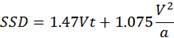Figure 69. Equation. Available SSD.

Where:

SSD = available stopping sight distance (ft (m)).
V = inferred design speed (mph (km/h)).
t = perception-reaction time (2.5 s).
a = deceleration rate (11.2 ft/s2 (3.4 m/s2)).

Substituting the given information to the SSD equation becomes figure 70.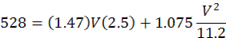Figure 70. Equation. Using available SSD equation to solve for inferred design speed.

Solving for inferred design speed using the quadratic equation, the inferred design speed is V = 57.46 mph (92.5 km/h).

### Length of Vertical Curve

The following vertical alignment design equations (figure 71 though figure 74) can be used to calculate inferred design speed based on the length of vertical curve.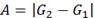Figure 71. Equation. Algebraic difference in grades.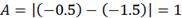Figure 72. Equation. Inputting given information to solve for algebraic difference in grades.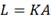Figure 73. Equation. Length of vertical curve.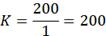Figure 74. Equation. Inputting given information to solve for length of vertical curve.

Where K is the rate of vertical curvature (ft/percent difference in grades (m/percent difference in grades)).

Table 3-36 in the Green Book can be used to determine the minimum SSD and design speed for a given rate of vertical curvature. (AASHTO 2011) Using a rate of vertical curvature of 200 and interpolating the design speed data shown in table 3-36, the following values are determined: SSD = 800.08 ft (243.9 m) and design speed = 73.89 mph (118.9 km/h).

### Radius of Curvature and Superelevation

The inferred design speed can be calculated for combinations of horizontal curvature and superelevation. The following equation (figure 75) from the Green Book (equation 3-9 in the Green Book) is referred to as the point-mass model, and it can be used to determine the inferred design speed based on the radius of curve-superelevation combination.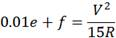Figure 75. Equation. Point-mass model to determine inferred design speed. (AASHTO 2011)

Both the speed and side-friction demand factor are unknown. Rearranging the point-mass model to solve for the side-friction demand factor produces the following equations (figure 76 through figure 78):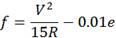Figure 76. Equation. Point-mass model to solve for the side-friction demand factor.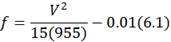Figure 77. Equation. Inputting given information into the point-mass model.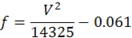Figure 78. Equation. Reducing inputted information in the point-mass model.

A trial-and-error process, using various design speeds and the information shown in table 3-7 from the Green Book, can be used to solve for the side-friction demand factor that does not exceed the maximum side-friction demand factor for a given design speed. (AASHTO 2011) Several iterations of this process will likely occur to obtain the inferred design speed.

First, a design speed of 50 mph (80.5 km/h) was used with the given superelevation of 6.1 percent. From table 3-7 in the Green Book, a design speed of 50 mph (80.5 km/h) has a maximum friction value of 0.14. The values were inputted into the equation (figure 79) to determine if the calculated friction value exceeded the given value.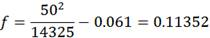Figure 79. Equation. Calculating the side-friction demand factor for a design speed of 50 mph (80.5 km/h).

The f value of 0.114 is smaller than the maximum f value of 0.14; therefore, a larger inferred design speed is considered.

The second iteration applied a design speed of 55 mph (88.5 km/h) with the given superelevation of 6.1 percent. From table 3-7 in the Green Book, the maximum friction factor for an inferred design speed of 55 mph (88.5 km/h) is 0.13 (figure 80).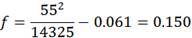Figure 80. Equation. Calculating the side-friction demand factor for a design speed of 55 mph (88.5 km/h).

The f value of 0.150 exceeds the maximum friction value of 0.13; therefore, a smaller inferred design speed should be considered.

A third iteration was completed using a design speed of 53 mph (85.3 km/h) with the given superelevation of 6.1 percent (figure 81). Interpolating from table 3-7 in the Green Book produces a maximum friction factor of 0.134 for an inferred design speed of 53 mph (85.3 km/h).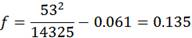Figure 81. Equation. Calculating the side-friction demand factor for a design speed of 53 mph (85.3 km/h).

The f value of 0.135 exceeds the maximum friction value of 0.134; therefore, a smaller inferred design speed should be considered.

A fourth iteration was completed using a design speed of 52 mph (83.7 km/h) with the given superelevation of 6.1 percent (figure 82). Interpolation from table 3-7 in the Green Book produces a maximum side friction factor of 0.136 for an inferred design speed of 52 mph (83.7 km/h).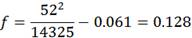Figure 82. Equation. Calculating the side-friction demand factor for a design speed of 52 mph (83.7 km/h).

The f value of 0.128 does not exceed maximum friction value of 0.136; therefore, the inferred design speed for the horizontal curve is 52 mph (83.7 km/h).

### HSO

The equation for the HSO can be used to calculate the radius of curvature, which can then be substituted into the horizontal curve equation to determine an inferred design speed. The following equation (figure 83) from the Green Book (equation 3-36 in the Green Book) is used for this purpose.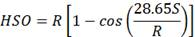Figure 83. Equation. HSO. (AASHTO 2011)

Where:

S = stopping sight distance (ft (m)).
R = radius of curve (ft (m)).

Substituting the given information produces figure 84.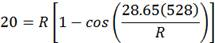Figure 84. Equation. Using HSO equation to solve for radius of curve.

Where R is 1739.31 ft (530.1 m).

Using the horizontal curve equation for figure 85 through figure 87 as follows:Figure 85. Equation. Point-mass model to determine inferred design speed.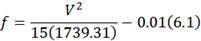Figure 86. Equation. Inputting given information into the point-mass model (step 1).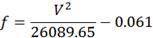Figure 87. Equation. Inputting given information into the point-mass model (step 2).

First, a trial design speed of 70 mph was used with a given superelevation of 6.1 percent. From table 3-7 in the Green Book, the maximum friction for a design speed of 70 mph (112.7 km/h) is 0.10 (figure 88).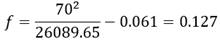Figure 88. Equation. Calculating the side-friction demand factor for a design speed of 70 mph (112.7 km/h). (AASHTO 2011)

The f value of 0.127 exceeds the maximum friction value of 0.10; therefore, a lower inferred design speed is considered.

The second iteration considered a design speed of 67 mph (107.8 km/h) with the given superelevation of 6.1 percent. From table 3-7 in the Green Book, the maximum friction value for a design speed of 67 mph (107.8 km/h) is 0.106 (figure 89).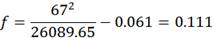Figure 89. Equation. Calculating the side-friction demand factor for a design speed of 67 mph (107.8 km/h). (AASHTO 2011)

The f value of 0.111 exceeds the maximum friction value of 0.106; therefore, a lower inferred design speed is considered.

The third iteration used a design speed of 66 mph with the given superelevation of 6.1 percent. From table 3-7 in the Green Book, the maximum friction value for a design speed of 66 mph (106.2 km/h) is 0.108 (figure 90).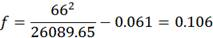Figure 90. Equation. Calculating the side-friction demand factor for a design speed of 66 mph (106.2 km/h). (AASHTO 2011)

The f value of 0.106 does not exceed the maximum friction value of 0.108; therefore, the inferred design speed for the horizontal curve using the HSO is 66 mph (106.2 km/h).

### Conclusion

The inferred design speed for this example site, which includes an overlapping horizontal and vertical curve, is the lowest among the values computed for each speed-influencing factor. As such, the horizontal curve radius-superelevation combination, with an inferred design speed of 52 mph (83.7 km/h), is the controlling geometric design feature.